## 1.1. 符号说明

 - $P = \lbrace I_i^{+}, I_j^{+}\rbrace$，训练数据集构建的全部 positive 图片对，$I_i^{+}$ 和 $I_j^{+}$ 是相同标签(label)或者相似标签的图片；

 - $N = \lbrace I_i^{-}, I_j^{-}\rbrace$，训练数据集构建的全部 negative 图片对，$I_i^{-}$ 和 $I_j^{-}$ 是不同标签(label)或者不相关标签的图片；

 - $G_k$，第 $k$ 个子网络模块；假设共 $K$ 个网络模块，$G_1$ 的网络输入是图片，而$G_k, k>1$ 的其它模块的输入是前一个模块的输出；$K$ 个网络模块级联地组成前馈网络；$K$ 个模型分别对应 $K$ 个不同深度的子网络；

 - $\lbrace o_{i,k}^{+}, o_{j,k}^{+}\rbrace$， 网络 $G_k$ 对于 positive 样本对 $\lbrace I_i^{+}, I_j^{+}\rbrace$ 计算的输出；

 - $\lbrace o_{i,k}^{-}, o_{j,k}^{-}\rbrace$， 网络 $G_k$ 对于 negative 样本对 $\lbrace I_i^{-}, I_j^{-}\rbrace$ 计算的输出；

 - $F_k$，第 $k$ 个变换函数，将 $o_k$ 转换为低维特征向量 $f_k$，以进行距离计算；

 - $\lbrace f_{i,k}^{+}, _{j,k}^{+}\rbrace$，$F_k$ 对于 positive 样本对 $\lbrace I_i^{+}, I_j^{+}\rbrace$ 计算的第 $k$ 个特征向量；

 - $\lbrace f_{i,k}^{-}, _{j,k}^{-}\rbrace$，$F_k$ 对于 negative 样本对 $\lbrace I_i^{-}, I_j^{-}\rbrace$ 计算的第 $k$ 个特征向量；

## 1.2. HDC 框架图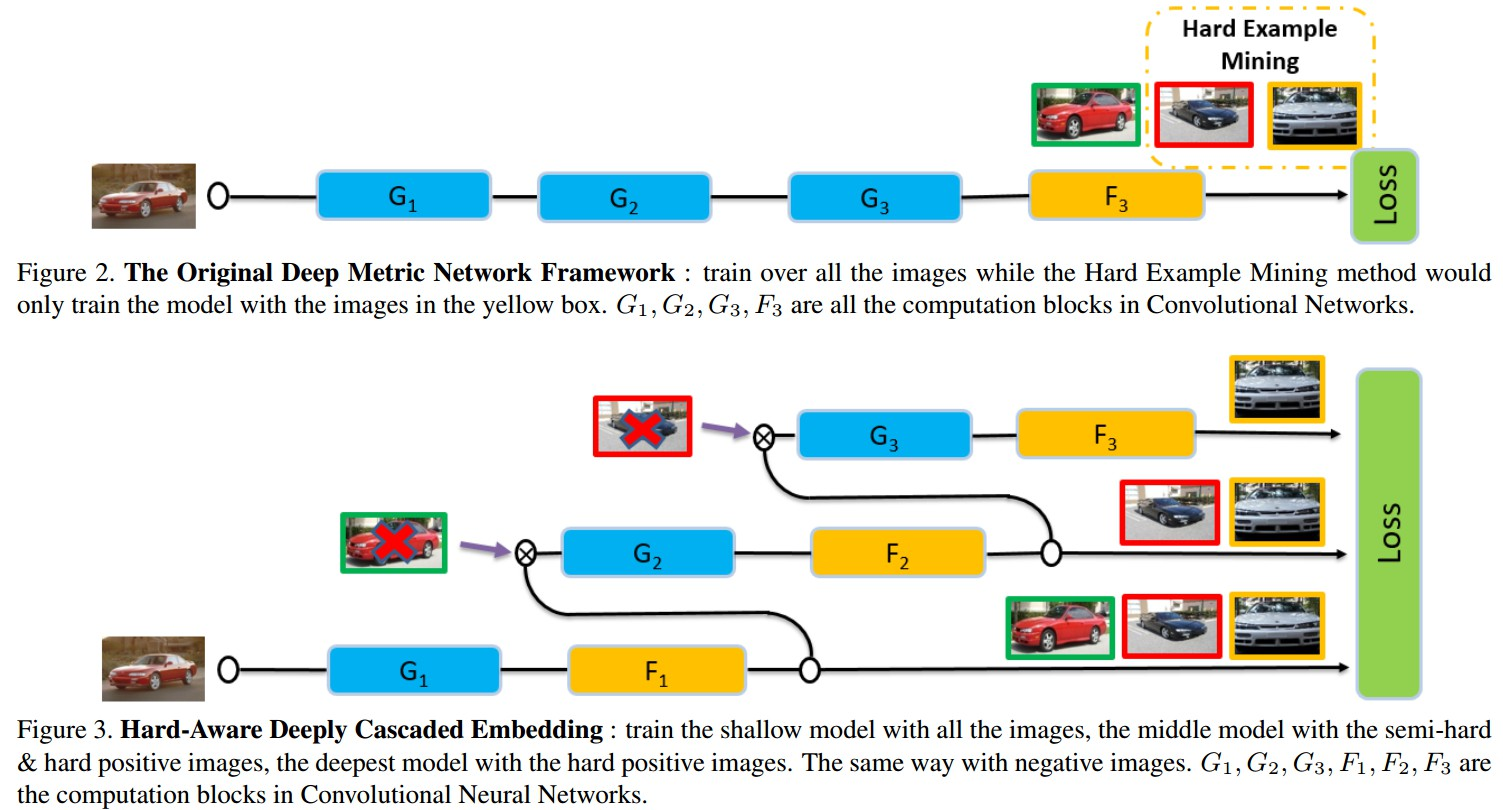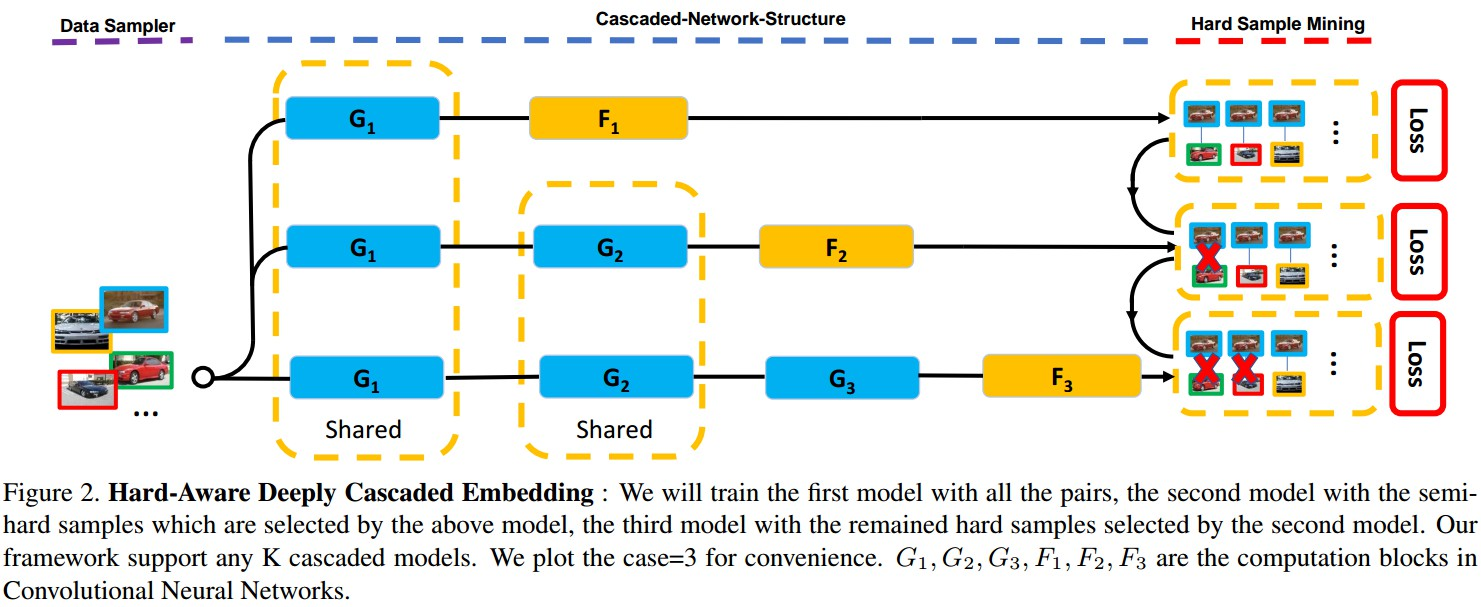Figure 2. HDC Embedding. 对所有的样本对训练模型 $G_1$，基于模型 $G_1$ 选择的 semi-hard samples 训练模型 $G_2$，基于模型 $G_2$ 选择的剩余的 hard samples 训练模型 $G_3$. $F_1, F_2, F_3$ 分别为 $G_1, G_2, G_3$ 的特征变换.

### 1.2.1. 前向计算

$$\lbrace o_{i, 1}, o_{j, 1}\rbrace = G_1 \circ \lbrace I_i, I_j\rbrace$$

$$\lbrace f_{i,1}, f_{j,1}\rbrace = F_1 \circ \lbrace o_{i, 1}, o_{j, 1}\rbrace$$

$$\lbrace o_{i, k}, o_{j, k}\rbrace = G_k \circ \lbrace o_{i, k-1}, o_{j, k-1}\rbrace$$

$$\lbrace f_{i,k}, f_{j, k}\rbrace = F_k \circ \lbrace o_{i, k}, o_{j, k}\rbrace$$

$$L_k = \sum _{(i, j) \in P_k} L_{k}^{+}(i, j) + \sum _{(i, j) \in N_k} L_{k}^{-}(i, j)$$

HDC 的最终 Loss 函数：

$$L = \sum_{k=1} ^{K} \lambda_k L_k$$

### 1.2.2. 梯度计算

Loss 关于模型 $G_k$ 的梯度计算：

$$\frac{\partial {L} }{\partial{G_k} } = \sum_{l=k}^K \lambda_l \frac{\partial{L_l}}{\partial{G_k}}$$

Loss 关于变换 $F_k$ 的梯度计算：

$$\frac{\partial {L} }{\partial{F_k} } =\lambda_k \frac{\partial{L_l}}{\partial{F_k}}$$

### 1.2.3. 对比损失

loss 函数- contrastive loss

Contrastive Loss 是使 positive 样本对和距离小于边缘参数的 negative 样本对间的距离尽可能大.

$$L^{+}(i, j) = D(f_i^{+}, f_j^{+})$$

$$L^{-}(i, j) = max\lbrace 0, M - D(f_i^{-}, f_j^{-})\rbrace$$

$D(f_i, f_j)$ - 两个 L2-normalized 特征向量 $f_i$ 和 $f_j$ 间的 Euclidean 距离.

$M$ - 边缘参数(margin)

$$L_k = \sum_{(i,j) \in P_k} D(f_{i, k}^{+}, f_{j, k}^{+}) + \sum_{(i, j)\in N_k)} max\lbrace 0, M - D (f_{i, k}^{-}, f_{j, k}^{-})\rbrace$$

## 1.3. Hard Example 的选择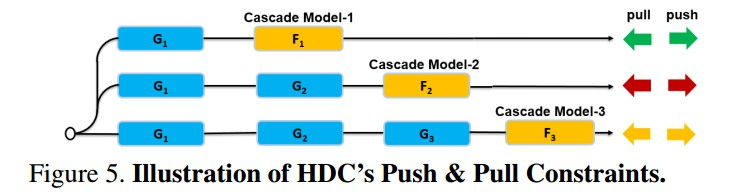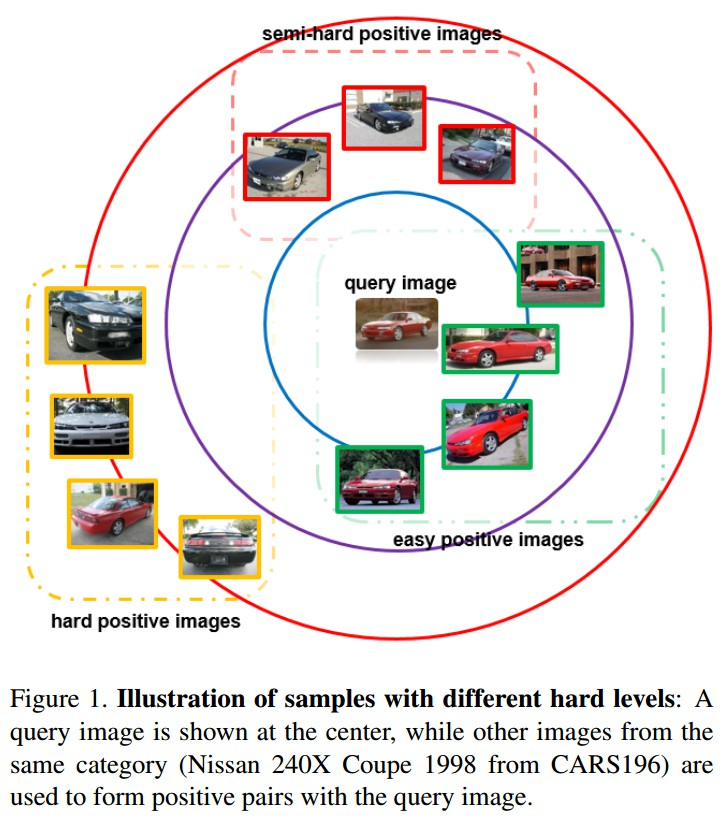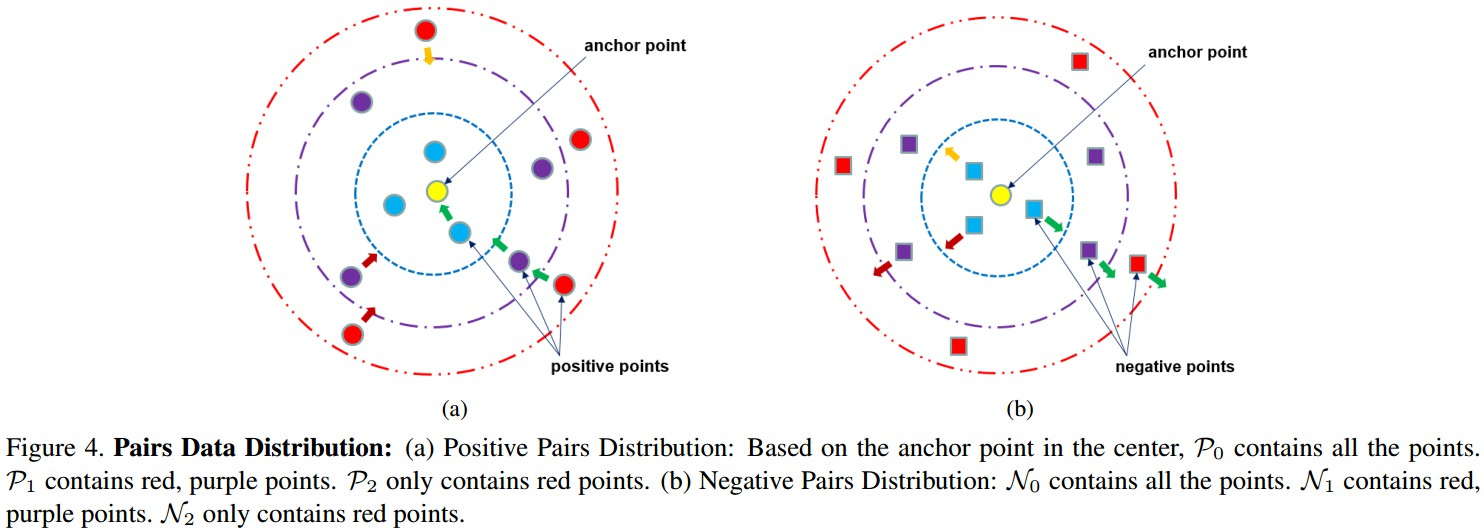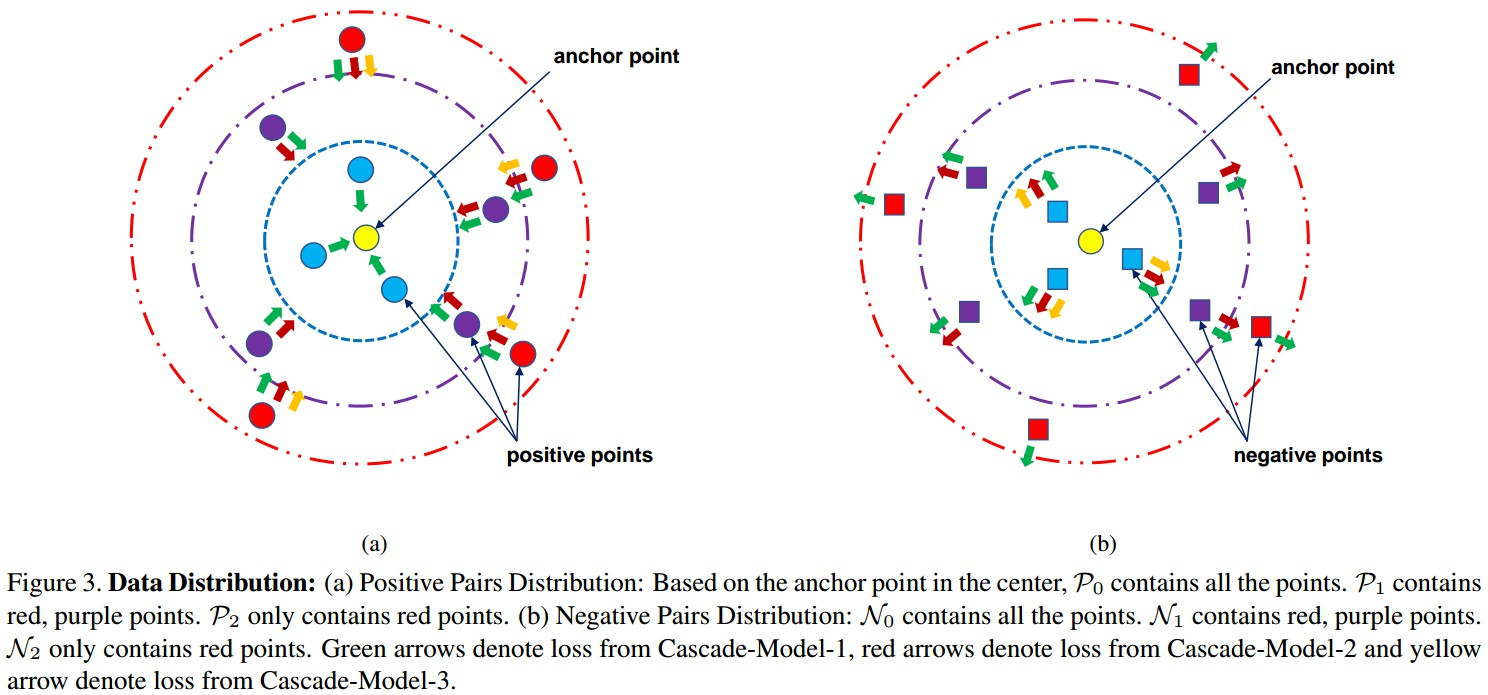Figure 3. 数据分布.

 - Positive 样本对分布：基于中心的 anchor 点，$P_0$ 包含所有的点，$P_1$ 包含红色、紫色的点，$P_2$ 只包含红色的点.

 - Negative 样本对分布： $N_0$ 包含所有的点，$N_1$ 包含红色、紫色的点；$N_2$ 只包含红色的点.

Cascade-Model-1 对 $P_0$ 和 $N_0$ 的所有样本对进行 forward，并尝试将所有的 positive 点 push 靠近到 anchor 点，同时将所有的 negative 点 push 远离 anchor 点； 根据 loss 值选择 hard samples，形成 $P_1$ 和 $N_1$(即，第2个和第3个虚线圆内的点). 类似地， Cascade-Model-2 得到 $P_2$ 和 $N_2$(即，第3个虚线圆内的点).

## 1.4 HDC 实现细节

 - 输入： 训练数据集 $\lbrace I_i\rbrace _{i=1}^N$

 - for $t = 1; t < N; t++$ do

 - xxxx 根据类似于 lifted structured feature embedding 的方法，采样 mini-batch 的训练图片；并根据[Learning a metric embedding for face recognition using the multibatch method]() 的方法对 mini-batch 内的训练图片初始化 $P_0$ 和 $N_0$.

- xxxx for $k = 1; k \leq K; k++$ do

 - xxxxxxxx 对样本集 $P_{k-1}$ 和 $N_{k-1}$ 内的所有图片进行 forward 到第 $k$ 个模型，以根据下面公式计算特征：

$\lbrace o_{i, k}, o_{j, k}\rbrace = G_k \circ \lbrace o_{i, k-1}, o_{j, k-1}\rbrace$

$\lbrace f_{i,k}, f_{j, k}\rbrace = F_k \circ \lbrace o_{i, k}, o_{j, k}\rbrace$

 - xxxxxxxx 根据下面的公式，计算 mini-batch 内全部样本对的losses：

$L^{+}(i, j) = D(f_i^{+}, f_j^{+})$

$L^{-}(i, j) = max\lbrace 0, M - D(f_i^{-}, f_j^{-})\rbrace$

 - xxxxxxxx 根据 1.3 中 hard examples 选择方法，选择 hard 样本对，得到 $P_k$ 和 $N_k$.

 - xxxxxxxx 根据梯度计算公式，对 $P_k$ 和 $N_k$ 内的所有样本对进行 backward 和更新：

$\frac{\partial {L} }{\partial{G_k} } = \sum_{l=k}^K \lambda_l \frac{\partial{L_l}}{\partial{G_k}}$

$\frac{\partial {L} }{\partial{F_k} } =\lambda_k \frac{\partial{L_l}}{\partial{F_k}}$

 - xxxx end for

 - end for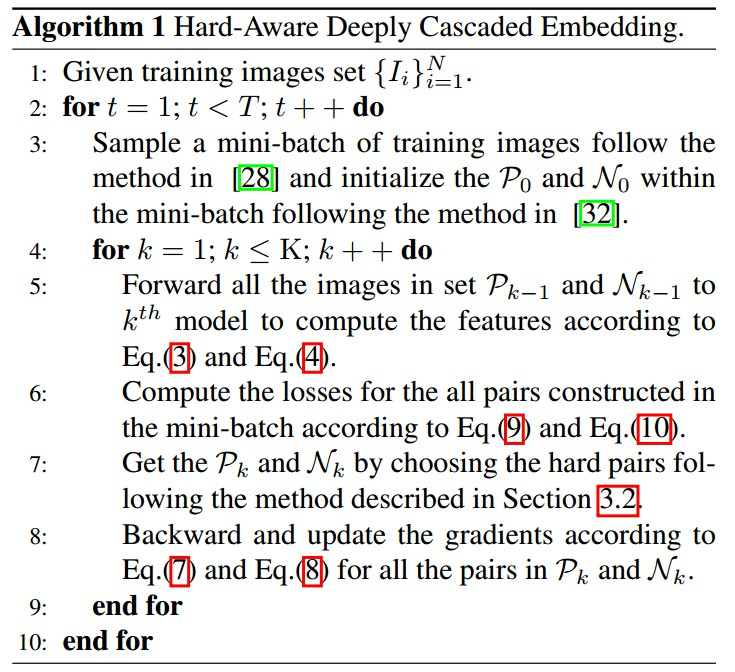## 2. Experiments

 - CARS196 dataset

196 类 cars，16185 张图片，前 98 类(8054张图片)作训练，其余 98 类(8131张图片) 作测试.

 - CUB-200-2011 dataset

200 类 birds，11788 张图片，前 100 类(5864 张图片)作训练，其余的(5924 张图片) 作测试.

 - Stanford Online Products dataset

22634 类 products，120053 张图片，11318 类(59551 张图片) 作训练，其余的 11316 类(共 60502 张图片)作测试.

 - In-shop Clothes Retrieval dataset
DeepFashion，11735 类 clothes，54642 张图片，从中筛选 7982 类(52712 张图片) 作训练和测试. 3997 类(25882 张图片)作训练，3985类(28760 张图片)作测试.

 - VehicleID dataset

26267 类 vehicles，221763 张图片，13134 类(110178 张图片)作训练，13133 类(111585 张图片)作测试.

### 2.2. Results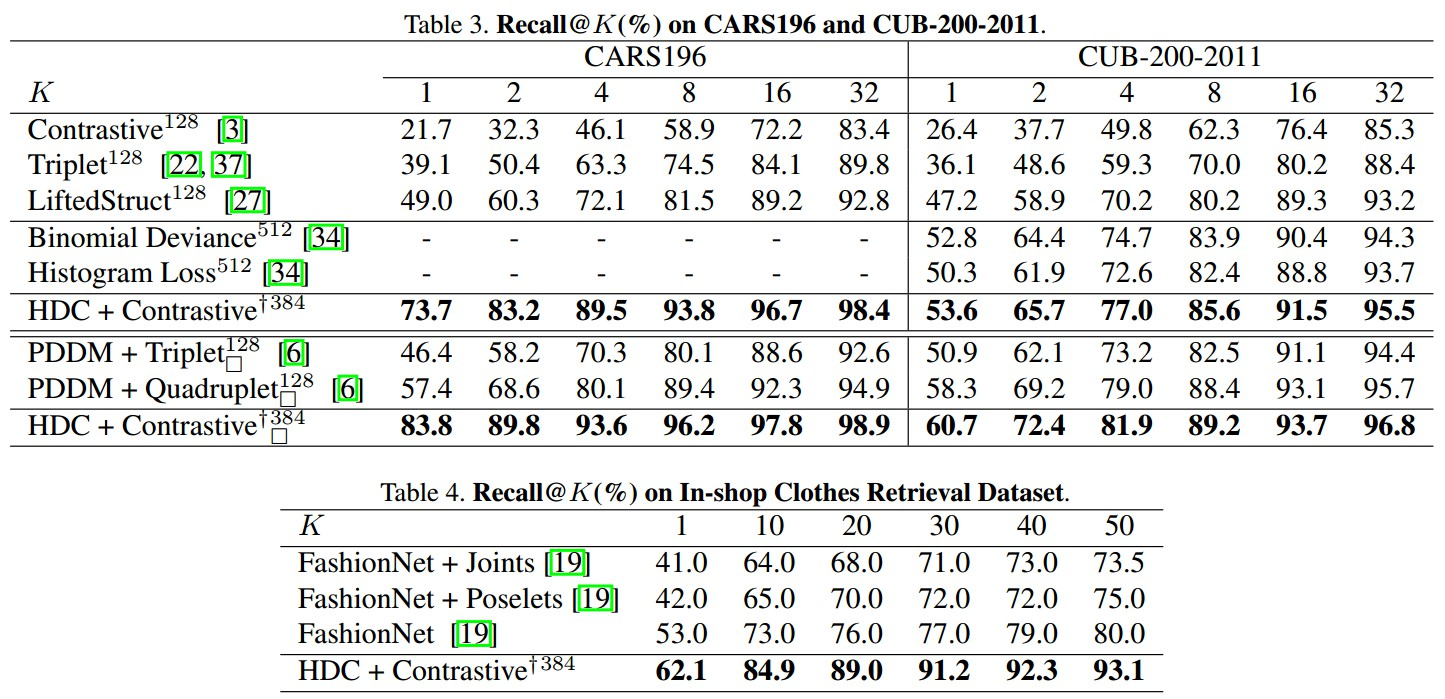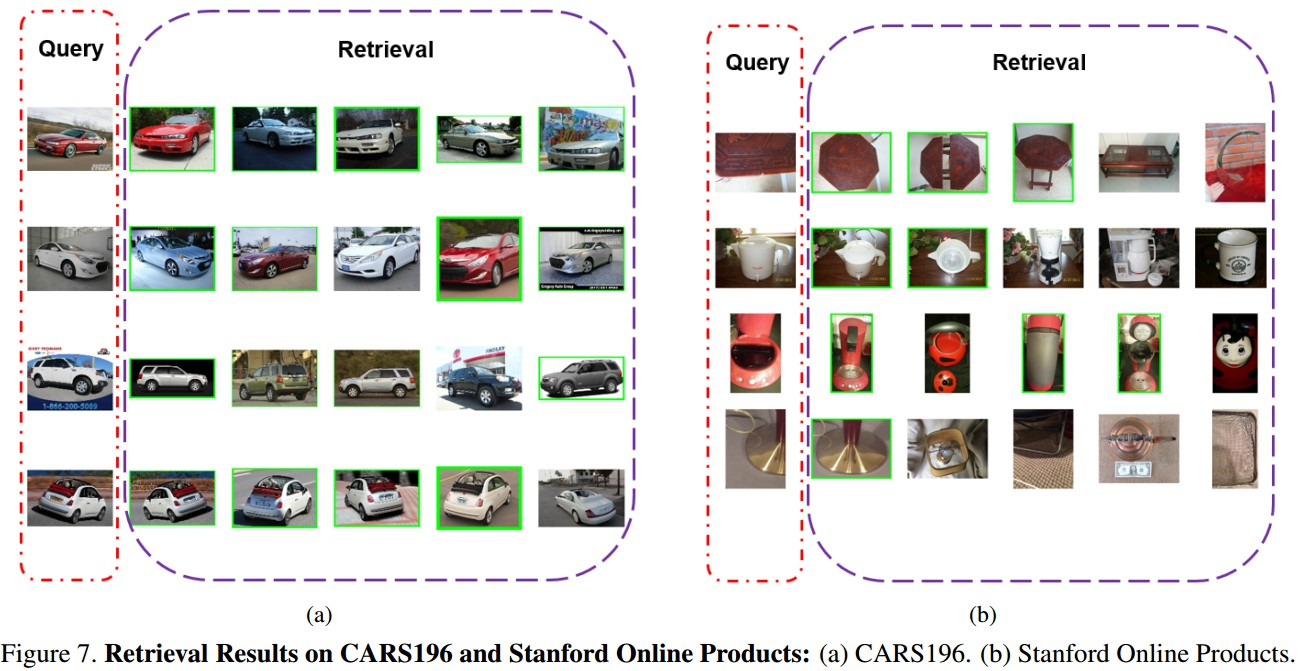Last modification：April 13th, 2021 at 01:46 pm